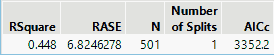Publication date: 11/29/2021

## Informative Missing

The Informative Missing option enables informative treatment of missing values on the predictors. The model that is fit is deterministic. The Informative Missing option is found on the launch window and is selected by default. When the Informative Missing option is selected, categorical and continuous predictors are handled differently:

Rows containing missing values for a categorical predictor are entered into the analysis as a separate level of the variable.

Rows containing missing values for a continuous predictor are assigned to a split as follows: The values of the continuous predictor are sorted. Missing rows are first considered to be on the low end of the sorted values. All splits are constructed. The missing rows are then considered to be on the high end of the sorted values. Again, all splits are constructed. The optimal split is determined using the LogWorth criterion. For further splits on the given predictor, the algorithm commits the missing rows to high or low values, as determined by the first split induced by that predictor.

If the Informative Missing option is not selected, the missing values are handled as follows:

When a predictor with missing values is used as a splitting variable, each row with a missing value on that predictor is randomly assigned to one of the two sides of the split.

The first time a predictor with missing values is used as a splitting variable an Imputes column is added to the Summary Report showing the number of imputations. As additional imputations are made, the Imputes column updates (Figure 4.15), where five imputations were performed.

Note: The number of Imputes can be greater than the number of rows that contain missing values. The imputation occurs at each split. A row with missing values can be randomly assigned multiple times. Each time a row is randomly assigned it increments the imputation count.

Figure 4.15 Impute Message in Summary Report